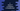# C program isxdigit method example to check if a character is hexadecimal## C program isxdigit method example to check if a character is hexadecimal:

The isxdigit() function is defined in the ctype.h header file and this function checks if a character is a hexadecimal character or not.

The hexadecimal number system is a base-16 number system and to represent a number in hexadecimal, 0 to 9 and a to f or A to F characters are used.

So, isxdigit() function will detect any of these character as hexadecimal.

### Definition of isxdigit:

The isxdigit function is defined as like below:

``int isxdigit(int d)``

This method takes a single character as its parameter. It converts that character to integer internally.

It checks if the character is a hexadecimal character or not.

#### Return value of isxdigit:

It returns a non-zero value if the character is a hexadecimal character. Else, it returns 0.

This function is defined in the ctype.h header file. We need to include it to use it in a C program.

### Example 1: Check if all characters in an array are hexadecimal or not:

Let’s check if all characters in an array are hexadecimal or not by using the isxdigit function. We will create an array with some characters and iterate over the character of the array one by one. For each character, it will use isxdigit to check if the character is hexadecimal or not and print a result.

We will use a for loop to iterate through the characters of the array. Inside the loop, it will use isxdigit to check each character.

Below is the complete program:

``````#include <stdio.h>
#include <ctype.h>

int main()
{
int arr[] = {'a', 'x', 'f', '0', '1', '5', '@', '&', 'A', 'F', 'G'};

for (int i = 0; i < 11; i++)
{
if (isxdigit(arr[i]))
{
printf("%c is a hexadecimal character\n", arr[i]);
}
else
{
printf("%c is not a hexadecimal character\n", arr[i]);
}
}

return 0;
}``````

In this program,

• arr is an array of different characters.
• The for loop is running from i = 0 to i = 10.
• For each iteration, it uses isxdigit to check if the current character is a hexadecimal character or not.
• Based on this value, it prints a message to the user.

If you run this program, it will print:

``````a is a hexadecimal character
x is not a hexadecimal character
@ is not a hexadecimal character
& is not a hexadecimal character
G is not a hexadecimal character``````

### Example 2: Check if a user input character is hexadecimal or not:

Let’s write another program that takes a character as the input and prints a message if it is hexadecimal or not.

``````#include <stdio.h>
#include <ctype.h>

int main()
{
char c;
printf("Enter a character: ");
scanf("%c", &c);

if (isxdigit(c))
{
printf("%c is a hexadecimal character\n", c);
}
else
{
printf("%c is not a hexadecimal character\n", c);
}

return 0;
}``````

It is similar to the above program. It reads a character as input from the user and stores the value in c. Based on the return value of isxdigit, it prints a message.

It will give output as like below:

``````Enter a character: c